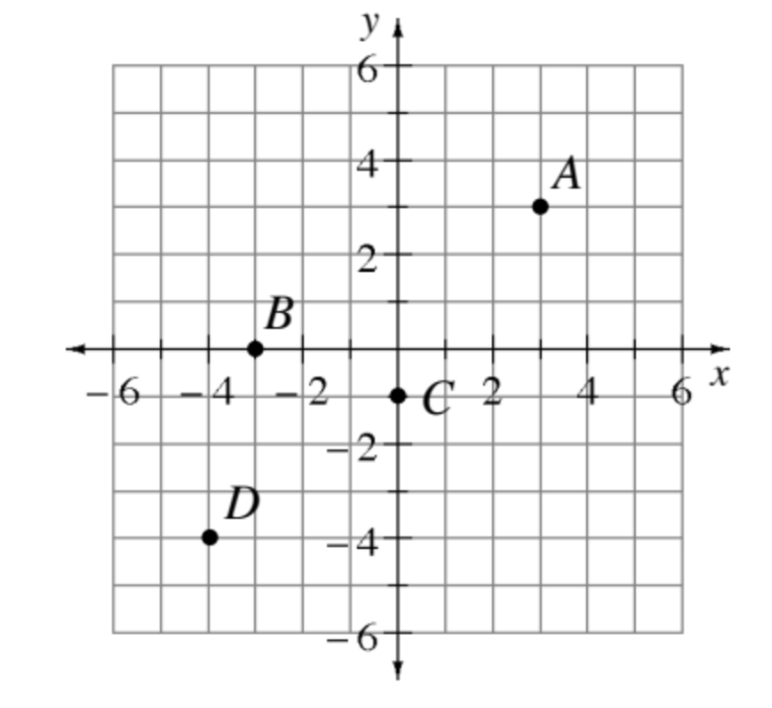### Home > PC3 > Chapter 11 > Lesson 11.2.5 > Problem11-145

11-145.In the diagram at right, points are plotted on a Cartesian grid. What are the polar coordinates of these points?

Each point should be written in the form $(r, θ)$, where $r$ is the distance from the point to the origin and $θ$ is the angle of rotation measured counterclockwise from the positive $x$-axis.

For points $A$ and $D$, draw a segment from the point to the origin. Use the Pythagorean Theorem to determine r and inverse tangent to determine $θ$.